# Exercise 7.1 Cubes and Cube Roots- NCERT Solutions Class 8

## Chapter 7 Ex.7.1 Question 1

Which of the following numbers are not perfect cubes?

(i) $$216$$

(ii) $$128$$

(iii) $$1000$$

(iv) $$100$$

(v) $$46656$$

### Solution

What is unknown?

To find the numbers which are not perfect cubes.

Reasoning:

A number is a prefect cube only when each factor in the prime factorization is grouped in triples.

Steps:

(i)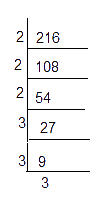\begin{align}216 &= {2 \times 2 \times 2} \times {3 \times 3 \times 3} \\&= {2^3} \times {3^3}\\& ={{(2\times 3)}^{3}} \\& ={{6}^{3}} \\\end{align}

$$\therefore 216$$ is a perfect cube

(ii)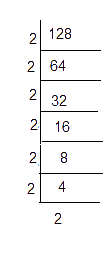\begin{align}128 = 2 \times 2 \times 2 \times 2 \times 2 \times 2 \times 2 \end{align}

$$128 = {2^3} \times {2^3} \times 2$$ one of the $$2$$ is not grouped in triples

$$\therefore \;128$$ is not a perfect cube

(iii)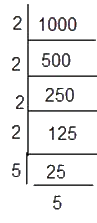\begin{align}1000 &= 2 \times 2 \times 2 \times 5 \times 5 \times 5\\ 1000 &= {2^3} \times {5^3}\end{align}

$$\therefore\;1000$$ is a perfect cube

(iv)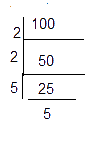\begin{align}100&= 2 \times 2 \times 5 \times 5\\&= {2^2} \times {5^2}\end{align}

Both $$2$$ and $$5$$ are not grouped in triples

$$\therefore \;100$$ is not a perfect cube.

(iv)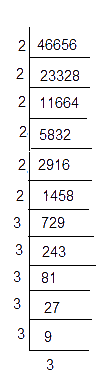\begin{align}46656 &= \left(\begin{array} \ \underline {2 \times 2 \times 2} \times \underline {2 \times 2 \times 2} \times \\ \underline {3 \times 3 \times 3} \times \underline {3 \times 3 \times 3}\end{array} \right) \\ &= {2^3} \times {2^3} \times {3^3} \times {3^3} \end{align}

$$\therefore\;46656$$ is a perfect cube

## Chapter 7 Ex.7.1 Question 2

Find the smallest number by which each of the following numbers must be multiplied to obtain a perfect cube.

(i) $$243$$

(ii) $$256$$

(iii) $$72$$

(iv) $$675$$

(v) $$100$$

### Solution

What is unknown?

To find the smallest number by which the given number must be multiplied to obtain a perfect cube.

Reasoning:

A number is a perfect cube only when each factor in the prime factorization is grouped in triples.Using this concept, the smallest number can be identified.

Steps:

(i)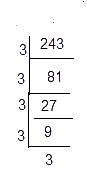\begin{align}243 &= \underline {3 \times 3 \times 3} \times 3 \times 3\\243 &= {3^3} \times 3\end{align}

Here, one of the $$3'\rm{s}$$ is  not a triplet.To make it as a triplet, we need to multiply by $$3$$

In that case,

\begin{align}243 \times 3 &= \underline {3 \times 3 \times 3} \times \underline {3 \times 3 \times 3} \\&= {3^3} \times {3^3} \\&= {9^3} = 729\end{align}

is a perfect cube.

Hence, the smallest natural number by which $$243$$ should be multiplied to make a perfect cube is $$3$$.

(ii)

\begin{align}256 &= \underline {2 \times 2 \times 2} \times \underline {2 \times 2 \times 2}\\& \times 2 \times 2\\256 &= {2^3} \times {2^3} \times {2^2}\end{align}

Here one of the $$2’\rm{s}$$ is not a triplet. To make it as a triplet, we need to multiply by $$2.$$

In that case,

\begin{align}256 \times 2 &= \underline {2 \times 2 \times 2} \times \underline {2 \times 2 \times 2} \\&\times \underline {2 \times 2 \times 2} \\&= {2^3} \times {2^3} \times {2^3} \\&= {8^3} = 512\end{align}

is a perfect cube.

Hence, the smallest natural number by which $$256$$ should be multiplied to make a perfect cube is $$2$$.

(iii)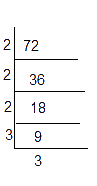\begin{align}72 &= \underline {2 \times 2 \times 2} \times 3 \times 3\\72 &= {2^3} \times {3^2}\end{align}

Here,on of the $$3$$'s is not a triplet. To make it as a triplet, we need to multiply by $$3.$$

In that case,

\begin{align}72 \times 3 &= \underline {2 \times 2 \times 2} \times \underline {3 \times 3 \times 3} \\&= {2^3} \times {3^3} \\&= {6^3} = 216\end{align}

is a perfect cube.

Hence, the smallest natural number by which 72 should be multiplied to make a perfect cube is 3.

(iv)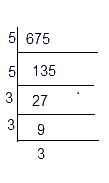\begin{align}675 &= 5 \times 5 \times \underline {3 \times 3 \times 3} \\675 &= {5^2} \times {3^3}\end{align}

Here, one of the $$5$$'s is not a triplet. To make it as a triplet, we need to multiply by $$5.$$

In that case,

\begin{align}675 &= \underline {5 \times 5 \times 5} \times \underline {3 \times 3 \times 3} \\&= {5^3} \times {3^3} \\&= {15^3} = 3375\end{align}

is a perfect cube.

Hence, the smallest natural number by which $$675$$ should be multiplied to make a perfect cube is $$5$$.

(v)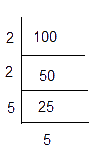\begin{align}100 &= 2 \times 2 \times 5 \times 5\\100 &= {2^2} \times {5^2}\end{align}

Here both the prime factors are not triplets. To make them triplets we need to multiply by one more  $$2$$ and $$5.$$

In that case,

\begin{align}100 &=\, \underline {2 \times 2 \times 2} \times \underline {5 \times 5 \times 5} \\&= {2^3} \times {5^3} \\&= {10^3} = 1000\end{align}

is a perfect cube.

Hence, the smallest natural number by which 100 should be multiplied to make a perfect cube is $$2 \times 5 = 10.$$

## Chapter 7 Ex.7.1 Question 3

Find the smallest number by which each of the following numbers must be divided to obtain a perfect cube.

(i) $$81$$

(ii) $$128$$

(iii) $$135$$

(iv) $$192$$

(v) $$704$$

### Solution

What is unknown?

To find the smallest number by which a given number must be divided to obtain a perfect cube.

Reasoning:

A number is a perfect cube only when each factor in the prime factorization is grouped in triples. Using this concept smallest number to be multiplied can be obtained.

(i)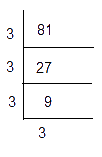\begin{align}81 &= \underline {3 \times 3 \times 3} \times 3\\81 &= {3^3} \times 3\end{align}

Here, the prime factor $$3$$ is not present as triples.

Hence, we divide by $$81$$ by $$3,$$ so that the obtained number becomes a perfect cube.

Thus,

\begin{align}81 \div 3 = 27 = {3^3}\end{align} is a perfect cube.

Hence the smallest number by which $$81$$ should be divided to make a perfect cube is $$3$$.

(ii)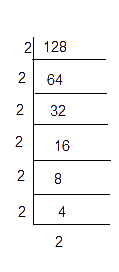\begin{align}128& = \underline {2 \times 2 \times 2} \times \underline {2 \times 2 \times 2} \times 2\\128 &= {2^3} \times {2^3} \times 2\end{align}

Here,the prime factors $$2$$ is not present as triples.

Hence, we divide $$128$$ by $$2,$$ so that the obtained number becomes a perfect cube.

\begin{align}128 \div 2 = 64 = {2^3} \times {2^3} = {4^3}\end{align} is a perfect cube.

Hence the smallest number by which $$128$$ should be divided to make a perfect cube is $$2$$.

(iii)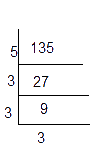\begin{align}135 &= 5 \times \underline {3 \times 3 \times 3} \\135 &= {5^1} \times {3^3}\end{align}

Here, the prime factors $$5$$ is not present as triples.

Hence, we divide $$135$$ by $$5,$$ so that the obtained number becomes a perfect cube.

\begin{align}135 \div 5 = 27 = {3^3}\end{align} is a perfect cube.

Hence the smallest number by which $$135$$ should be divided to make a perfect cube is $$5$$.

(iv)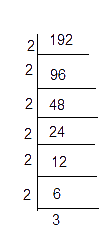\begin{align}192 &= \underline {2 \times 2 \times 2} \times \underline {2 \times 2 \times 2} \times 3\\192 &= {2^3} \times {2^3} \times 3\end{align}

Here,the prime factors $$3$$ is not present as triples.

Hence, we divide $$192$$  by $$3,$$ so that the obtained number becomes a perfect cube.

\begin{align}192 \div 3 = 64 = {2^3} \times {2^3} = {4^3}\end{align} is a perfect cube.

Hence the smallest number by which $$192$$ should be divided to make a perfect cube is $$3$$.

(v)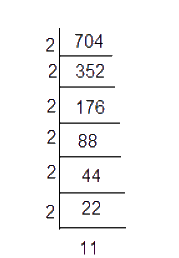\begin{align}704 &= \underline {2 \times 2 \times 2} \times \underline {2 \times 2 \times 2} \times 11\\704 &= {2^3} \times {2^3} \times 11\end{align}

Here, the prime factors $$11$$ is not present as triples.

Hence, we divide $$704$$ by $$11,$$ so that the obtained number becomes a perfect cube.

\begin{align}704 \div 11 = 64 = {2^3} \times {2^3} = {4^3}\end{align} is a perfect cube.

Hence the smallest number by which $$704$$ should be divided to make a perfect cube is $$11$$.

## Chapter 7 Ex.7.1 Question 4

Parikshit makes a cuboid of plasticine of sides $$5 \,\rm{cm},$$ $$2 \,\rm{cm,}$$ $$5\, \rm{cm.}$$ How many such cuboids will he need to form a cube?

### Solution

What is known?

Dimensions of cuboid $$5\;{\rm{cm}}\, \times\,2\;{\rm{cm}}\,\times \,5\;\rm{cm}.$$

What is unknown?

To find out the number of cuboids to form a cube.

Reasoning:

Number of cuboids required

\begin{align}=\frac{{{\text{Volume of cube}}}}{{{\text{Volume of cuboid}}}}\end{align}

Steps:

Volume of cuboid

\begin{align}&={\rm{length}}\! \times\! {\rm{breadth}} \\&\times {\rm{height}}\\ &= 5 \times 2 \times 5\\&= {5^2} \times {2^1}{\rm{c}}{{\rm{m}}^3}\end{align}

To make the volume of cuboid as a cube number we need to multiply it by $$5 \times 2 \times 2$$

\begin{align}&\text {Newly formed cube}\\&= {5^2} \times {2^1} \times 5\; \times 2 \times 2\\&= {5^3} \times {2^3}{\rm{c}}{{\rm{m}}^3}\end{align}

\begin{align}&\text {Number of cuboids required} \\&=\frac{5^{3} \times 2^{3}}{5^{2} \times 2}\\&=\frac{5 \times \not5 \times \not5 \times \not2 \times 2 \times 2}{\not 5 \times \not 5 \times \not2}\end{align}

$$\therefore \;$$ Number of cuboids required to make a cube $$=$$ \begin{align}5\times 2\times 2=20\end{align}

$$20$$ cuboids required.

Instant doubt clearing with Cuemath Advanced Math Program